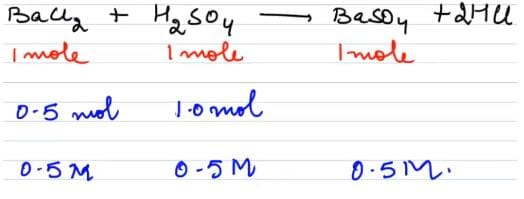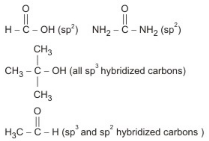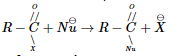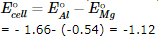JEE  >  BITSAT Chemistry Test - 5

# BITSAT Chemistry Test - 5

Test Description

## 40 Questions MCQ Test BITSAT Mock Tests Series & Past Year Papers | BITSAT Chemistry Test - 5

BITSAT Chemistry Test - 5 for JEE 2023 is part of BITSAT Mock Tests Series & Past Year Papers preparation. The BITSAT Chemistry Test - 5 questions and answers have been prepared according to the JEE exam syllabus.The BITSAT Chemistry Test - 5 MCQs are made for JEE 2023 Exam. Find important definitions, questions, notes, meanings, examples, exercises, MCQs and online tests for BITSAT Chemistry Test - 5 below.
Solutions of BITSAT Chemistry Test - 5 questions in English are available as part of our BITSAT Mock Tests Series & Past Year Papers for JEE & BITSAT Chemistry Test - 5 solutions in Hindi for BITSAT Mock Tests Series & Past Year Papers course. Download more important topics, notes, lectures and mock test series for JEE Exam by signing up for free. Attempt BITSAT Chemistry Test - 5 | 40 questions in 50 minutes | Mock test for JEE preparation | Free important questions MCQ to study BITSAT Mock Tests Series & Past Year Papers for JEE Exam | Download free PDF with solutions
 1 Crore+ students have signed up on EduRev. Have you?
BITSAT Chemistry Test - 5 - Question 1

### Which of the following cannot be made by using Williamson's synthesis?

BITSAT Chemistry Test - 5 - Question 2

### Catalyst used in Rosenmund reduction is

BITSAT Chemistry Test - 5 - Question 3

### Which of the following has more unpaired d-electrons?

BITSAT Chemistry Test - 5 - Question 4

If uncertainty in the position of electron is zero, the uncertainty in its momentum would be

BITSAT Chemistry Test - 5 - Question 5

The maximum amount of BaSO4 precipitated on mixing BaCl2 (0.5 M) with H2SO4 (1M) will correspond to

Detailed Solution for BITSAT Chemistry Test - 5 - Question 5BITSAT Chemistry Test - 5 - Question 6

2 g of oxygen contains number of atoms equal to that in

BITSAT Chemistry Test - 5 - Question 7

The compound with carbon uses only its sp3 hybrid orbitals for bond formation is

Detailed Solution for BITSAT Chemistry Test - 5 - Question 7BITSAT Chemistry Test - 5 - Question 8

Lipids are

BITSAT Chemistry Test - 5 - Question 9

A 'base-sugar phosphate' unit in nucleic acid is called

BITSAT Chemistry Test - 5 - Question 10

The reaction,is fastest when X is

BITSAT Chemistry Test - 5 - Question 11

The hybrid states of carbon in diamond, graphite and acetylene are respectively

BITSAT Chemistry Test - 5 - Question 12

The enthalpy of formation for C2H4(g),CO2(g) and H2O(l) at 25oC and 1 atm. pressure be 52,-394 and -286 kJ mol-1 respectively .The enthalpy of combustion of C2H4(g) will be

BITSAT Chemistry Test - 5 - Question 13

Elimination of bromine from 2- bromobutane results in the formation of

BITSAT Chemistry Test - 5 - Question 14

1 mole of N₂ and 2 moles of H₂ are allowed to react in a 1 dm3 vessel. At equilibrium 0.8 mole of NH₃ is formed. The concentration of H₂ in the vessel is

BITSAT Chemistry Test - 5 - Question 15

The rate of a reaction depends upon

BITSAT Chemistry Test - 5 - Question 16

The ionisation potential is lowest for the

Detailed Solution for BITSAT Chemistry Test - 5 - Question 16

In the periodic table, the ionisation potential is lowest for the alkali metals because the alkali metals have only one electron in the outermost s-orbital.

BITSAT Chemistry Test - 5 - Question 17

Hess's law deals with

BITSAT Chemistry Test - 5 - Question 18

BITSAT Chemistry Test - 5 - Question 19

The correct structure of Fe(CO)₅ is (Z = 26 for Fe)

BITSAT Chemistry Test - 5 - Question 20

Lanthanoids are 14 elements in the

BITSAT Chemistry Test - 5 - Question 21

In the transition series, as the atomic no. increases, the paramagnetism

BITSAT Chemistry Test - 5 - Question 22

The e.m.f. of a galvanic cell with electrode potentials of Al equal to -1.66 V and that of Mg equal to - 0.54 V is

Detailed Solution for BITSAT Chemistry Test - 5 - Question 22

E.M.F. can be positive only if oxidation takes place at Mg-electrode. But here the reduction reaction takes place.BITSAT Chemistry Test - 5 - Question 23

Which one of the following compounds will be most easily attacked by an electrophile ?

Detailed Solution for BITSAT Chemistry Test - 5 - Question 23

- NH2 is highly activating group

BITSAT Chemistry Test - 5 - Question 24

Which of the following has highest chlorine content?

BITSAT Chemistry Test - 5 - Question 25

When HCl gas is passed through a saturated solution of common salt, pure NaCl is precipitated because

BITSAT Chemistry Test - 5 - Question 26

In the keto-enol tautomerism of dicarbonyl compounds the enol form is preferred in contrast to the keto form, this is due to

BITSAT Chemistry Test - 5 - Question 27

Iodoethane reacts with sodium in ether to form

BITSAT Chemistry Test - 5 - Question 28

Which of the following is isoelectronic as well as has same structure as that of N₂O?

BITSAT Chemistry Test - 5 - Question 29

The nucleus of an atom consists of

BITSAT Chemistry Test - 5 - Question 30

BITSAT Chemistry Test - 5 - Question 31

Which of the following is not a characteristics of carbon?

BITSAT Chemistry Test - 5 - Question 32

Which of the following does not give a borax bead test?

BITSAT Chemistry Test - 5 - Question 33

Bio-gas and producer gas are made up of

BITSAT Chemistry Test - 5 - Question 34

Copolymer is

BITSAT Chemistry Test - 5 - Question 35

Glauber's salt is

BITSAT Chemistry Test - 5 - Question 36

White phosphorus reacts with caustic soda to produce PH₃ and NaH₂PO₄. The reaction is an example for

BITSAT Chemistry Test - 5 - Question 37

A sample of air contains only N₂, O₂ and H₂O. It is saturated with water vapours and the total pressure is 640 torr. The vapour pressure of water is 40 torr and the molar ratio of N₂:O₂: is 3:1. The partial pressure of N₂ in the sample is

Detailed Solution for BITSAT Chemistry Test - 5 - Question 37 Let the ratio of moles of N2 : O2 :H2O be 3:1:xPartial Presure of any gas = Mole fraction * Total PressurePartial Pressure of H2O vapour = 40 torr40 = Mole fraction of H2O * 640Mole fraction of H2O = 40/640x/(4+x) = 40/640x = 4/15N2 : O2 :H2O = 3 : 1 : 4/15= 45 : 15 : 4Partial Pressure of N2 = Mole fraction of N2 * 640= 45/(45+15+4) * 640 = 450 torr
BITSAT Chemistry Test - 5 - Question 38

2 gram of sulphur is completely burnt in oxygen to form SO2. In this reaction what is the volume of oxygen consumed at STP in litres

BITSAT Chemistry Test - 5 - Question 39

The ability of an ion to bring about coagulation of a given colloid depends on

BITSAT Chemistry Test - 5 - Question 40

What is the molarity of H₂SO₄ solution that has a density of 1.84 gm/cc at 35oC and contains 98% by weight?

## BITSAT Mock Tests Series & Past Year Papers

2 videos|15 docs|70 tests
 Use Code STAYHOME200 and get INR 200 additional OFF Use Coupon Code
Information about BITSAT Chemistry Test - 5 Page
In this test you can find the Exam questions for BITSAT Chemistry Test - 5 solved & explained in the simplest way possible. Besides giving Questions and answers for BITSAT Chemistry Test - 5, EduRev gives you an ample number of Online tests for practice

## BITSAT Mock Tests Series & Past Year Papers

2 videos|15 docs|70 tests